Get instant live expert help with Excel or Google Sheets“My Excelchat expert helped me in less than 20 minutes, saving me what would have been 5 hours of work!”

#### Post your problem and you’ll get expert help in seconds.

Your message must be at least 40 characters
Our professional experts are available now. Your privacy is guaranteed.

# How to Count Total Cells in a Range

We can count the total number of cells, rows, and column in a range by using the COLUMNS function and the ROWS function. The steps below will walk through the process.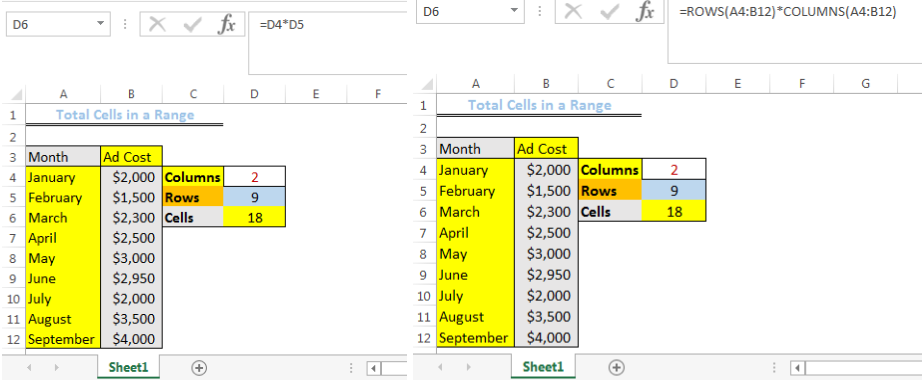Figure 1: Total number of Cells in the Range

## Formulato Count Total Cells in a Range

`=ROWS(range)*COLUMNS(range)`

## Setting up the Datato Count Total Cells in a Range

We will set up the data by imputing the values as shown in figure 2 into the various cells

• Cell A4 to Cell A12 will contain the Month
• Cell B4 to Cell B12 will contain the Ad cost for each month
• Cell C4, Cell C5, and Cell C6 will contain Columns, Rows, and Cells respectively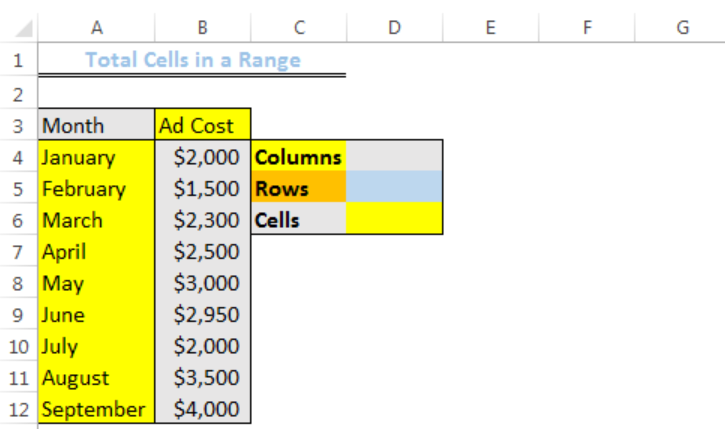Figure 2: Setting up the Data

## Counting the Total Columns in the Range (A4:B12)

We will input the formula below into Cell D4 to count the total number of columns in the range and press the enter key

`=COLUMNS(A4:B12)`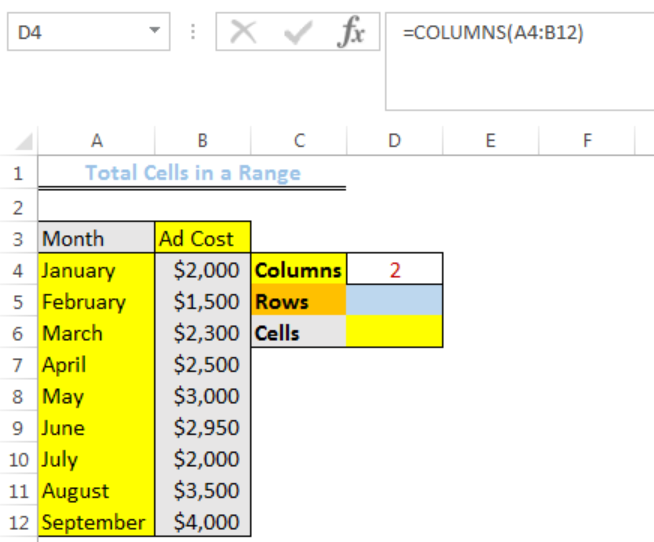Figure 3: Result for the Total number of Columns in the Range

## Counting the Total Rows in the Range (A4:B12)

We will input the formula below into Cell D5 to count the total number of rows in the range and press the enter key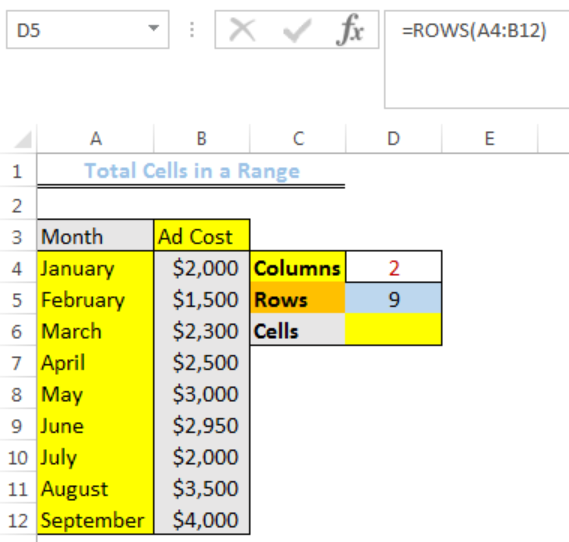Figure 4: Result for the Total number of Rows in the Range

## Counting the Total Cells in the Range (A4:B12)

We can find the total number of cells by multiplying the cell references of the number of columns and number of rows that we have determined. We will input the formula below into Cell D6 to count the total number of cells in the range and press the enter key

`=D4*D5`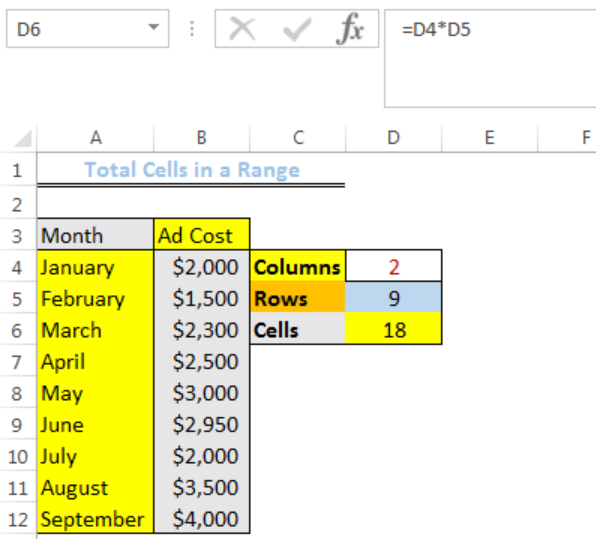Figure 5A: Result for the Total number of Cells in the Range

Alternatively, we can use the formula that combines the row and column functions. With this formula, there is no need of calculating the number of rows and columns independently. We will input the formula below into Cell D6 and press the enter key

`=ROWS(A4:B12)*COLUMNS(A4:B12)`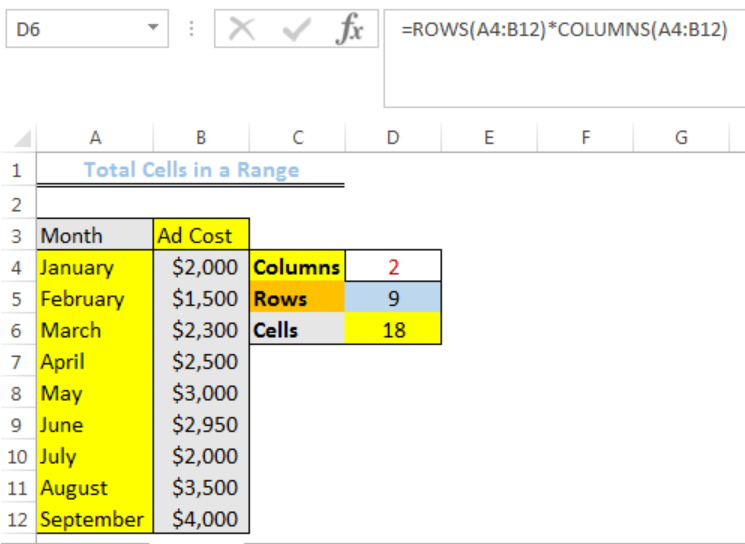Figure 5B: Result for the Total number of Cells in the Range

## Explanation

• ROWS Function

This function retrieves the total number of rows that are present in the range.

• COLUMNS Function

This function retrieves the total number of columns that are present in the range.

Once the value of both functions have been returned, the multiplication sign multiplies both values and returns the result as the total cells in the range.

## Instant Connection to an Expert through our Excelchat Service

Most of the time, the problem you will need to solve will be more complex than a simple application of a formula or function. If you want to save hours of research and frustration, try our live Excelchat service! Our Excel Experts are available 24/7 to answer any Excel question you may have. We guarantee a connection within 30 seconds and a customized solution within 20 minutes.

### Did this post not answer your question? Get a solution from connecting with the expert.Another blog reader asked this question today on Excelchat:
Solution examplesI need to create a pivot table that sums visits per day by provider: Date...Visits....Provider So, I can ask...how many visits did provider X have on day Y?
Solved by F. J. in 34 minscould someone breakdown this formula for me :D =ArrayFormula(IF(D\$5>=ROWS(B\$5:B5),INDEX(Sheet1!C\$6:C\$21,SMALL(IF(Sheet1!D\$6:D\$21=\$E\$5,ROW(Sheet1!C\$6:C\$21)-ROW(Sheet1!C\$6)+1),ROWS(B\$5:B5))),""))
Solved by C. C. in 40 minsDIVIDE NUMBER IN COLUMN A BY A FIXED NUMBER AND THEN SUBTRACT THAT TOTAL BY 1. HOW DO I COPY AND PASTE THIS FORMULA SO THAT IT APPLIES TO ALL OF THE ROWS?
Solved by A. U. in 40 mins## Subscribe to Excelchat.coAnother blog reader asked this question today on Excelchat: